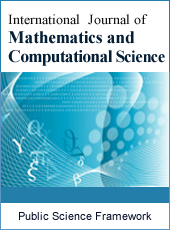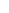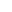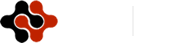International Journal of Mathematics and Computational Science
Articles Information
International Journal of Mathematics and Computational Science, Vol.1, No.5, Oct. 2015, Pub. Date: Jun. 24, 2015
Application of the GDQ Method to Vibration Analysis
 Ramzy M. Abumandour, Basic Engineering Science Department, Faculty of Engineering, Menofia University, Shebin El-Kom, Menofia, Egypt.
 M. H. Kamel, Engineering Mathematics and Physics Department, Faculty of Engineering, Cairo University, Giza, Egypt.
 M. M. Nassar, Engineering Mathematics and Physics Department, Faculty of Engineering, Cairo University, Giza, Egypt.
This paper study the vibration analysis using the differential quadrature method (DQM) which has very wide applications in the field of structural vibration of various elements such as beams, plates, cylindrical shells and tanks. One of the most advantages of the DQM is its simple forms for nonlinear formulations. In this paper, the free vibration of uniform and non-uniform beams resting on fluid layer under axial force under three sets of boundary conditions, that is, simply–simply supported (S–S), clamped–clamped supported (C–C) and clamped–simply supported (C–S) were studied using the generalized differential quadrature (GDQ). The proposed approach directly substitutes the boundary conditions into the governing equations (SBCGE). The approach of directly SBCGE is presented to overcome the drawbacks of previous approaches in treating the boundary conditions. The non-dimensional natural frequency and the normalized mode shapes of uniform and non-uniform beams were obtained. Results show good agreement with the previous analytical solutions. The effect of the varying cross section area on the vibration was studied. This work reflects the power of the DQM in solving non-uniform problems.
Uniform and Non-Uniform Beam, Vibration, Differential Quadrature Method
 Bellman, R. E., J. Casti, 1971. Differential quadrature and long-term integration. Journal of Mathematical Analysis and Applications, 34: 235–238.
 Bellman, R. E., B. G. Kashef and J. Casti, 1972. Differential quadrature: A technique for the rapid solution of non-linear partial differential equations. Journal of computational Physics 10: 40–52.
 Bert, C. W., Jang, S. K. and Striz A. G. 1988. Two new methods for analyzing free vibration of structure components. AIAA Journal, 26: 612–618.
 Bert, C., Wang, X. and Striz, A. G. 1993. Differential quadrature for static and free vibration analysis of anisotropic plates. International Journal of Solids Structures 30: 1737–1744.
 Bert, C., Wang, X. and Striz, A. G. 1994. static and free vibrational analysis of beams and plates by differential quadrature method. Acta Mechanica 102: 11–24.
 Blevins, R. D., 1984. Formulas for Natural Frequency and Mode Shapes. Malabur, Florida. Robert E. Krieger.
 Civan, F., and Sliepcevich, C. M., 1984. Differential quadrature for multi-dimensional problems. Journal of Mathematical Analysis Applied 101: 423–443.
 Civan, F., and Sliepcevich, C. M., 1983. Application of differential quadrature to transport processes. Journal of Mathematical Analysis Applied 93: 711–724.
 Du, H., Lim, M. K. and Lin, R. M., 1994. Application of Generalized Differential Quadrature to Structural problems. Journal of Sound and Vibrations 181: 279–293.
 Du, H., Lim, M. K. and Lin, R. M., 1995. Application of Generalized Differential Quadrature to Vibration Analysis. Journal of Sound and Vibrations 181: 279–293.
 Jang, S. K., Bert, C. W. and Striz A. G., 1989. Application of differential quadrature to static analysis of Structural components. International Journal of Numerical Methods in Engineering 28: 561–577.
 Quan, J. R., and Chang C. T., 1989. New insights in solving distributed system equations by the quadrature method-I, analysis. Journal of Computers and Chemical Engineering 13: 779–788.
 Qiang Guo and Zhong, H., 2004. Non-linear Vibration Analysis of Beams by a Spline-based differential quadrature. Journal of Sound and Vibration 269: 405–432.
 Shu, C. and Richards, B. E., 1990. High resolution of natural convection in a square cavity by generalized differential quadrature. Proceedings of 3rd International Conference on Advanced in numerical Methods in Engineering: Theory and Applications, Swansea, U.K 2: 978–985.
 Shu, C., 1991. Generalized differential-integral quadrature and application to the simulation of incompressible viscous flows including parallel computation. PhD thesis, University of Glasgow.
 Shu, C., 2000. Differential Quadrature and its Application in Engineering. Springer, Berlin.
 Wen, D., and Yu, Y., 1993a. Calculation and analysis of weighting coefficient matrices in differential quadrature method. Computational Engineering. Elsevier Oxford 157–162.
 Wen, D., and Yu, Y., 1993b: "Differential quadrature method for high order boundary value problems." In computational Engineering (Edited by Kwak, B. M. and Tanaka, M.): pp. 163–168. Elsevier Oxford.ISSN Print: 2381-7011
ISSN Online: 2381-702X
Current Issue:
Vol. 5, Issue 2, June Submit a Manuscript Join Editorial Board Join Reviewer Team
 About This Journal All Issues Open Access Indexing Payment Information Author Guidelines Review Process Publication Ethics Editorial Board Peer Reviewers600 ATLANTIC AVE, BOSTON,
MA 02210, USA+001-6179630233
JournalsJournal ListsAuthor GuidelinesPayment InformationSubmit a ManuscriptJoin as Editorial MembersJoin as ReviewersPrivacy NoticeTerms of ConditionsHelp Center